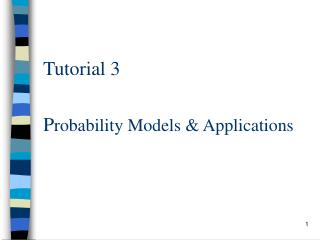DownloadDownload PresentationP robability Models & Applications

# P robability Models & Applications

Télécharger la présentation## P robability Models & Applications

- - - - - - - - - - - - - - - - - - - - - - - - - - - E N D - - - - - - - - - - - - - - - - - - - - - - - - - - -
##### Presentation Transcript

1. Probability Models & Applications Tutorial 3

2. Poisson Distribution • A random variable X, taking on one of the values 0, 1, 2, …, is said to be a Poisson random variable with parameter λ, if for some λ> 0,

3. Approximate Binomial by Poisson Distribution • Suppose X is a binomial random variable with parameter (n,p) and let λ = np.

4. Approximate Binomial by Poisson Distribution (con’t) • Now, for n large and p small, • Hence, for n large and p small,

5. Example 1 • Suppose that the probability that an item produced by a certain machine will be defective is 0.1. Find the probability that a sample of 10 items will contain at most 1 defective item. • Using Binomial dist,

6. Example 1 (con’t) • Using Poisson approximation,Now n = 10, p = 0.1, Let λ= 10x0.1 = 1 • P = P{X = 0} + P{X = 1}

7. Conditional Probability • Two events E and F, the conditional probability of E given F is defined, as long as P(F) > 0, by

8. Conditional Probability Mass Function • X and Y are discrete r.v., the conditional probability mass function of X given Y = y, is defined by

9. Example 2 • Suppose that p(x,y) , the joint probability mass function of X and Y, is given by p(1,1)=0.5, p(1,2)=0.1, p(2,1)=0.1, p(2,2)=0.3 Calculate the probability mass function of X given that Y=1. • We first note that

10. Example 2 (con’t) • Hence, • Similarly,

11. Conditional Probability Density Function • X and Y have a joint probability density function f(x,y), then the conditional probability density function of X, given Y=y, is defined for all values of y such that , by

12. Example 3 • Suppose the joint density of X and Y is Compute the conditional density function of X given Y=y, where 0<y<1.

13. Example 3 (con’t)

14. Matlab • 1) After logon to a workstation, type “matlab” to run the matlab program. • 2) How to program: i) Compute in the command prompt. ii) Use editor to edit the matlab file and same as .m extension. • 3) Type the file name to run the matlab file (e.g. filename mat.m, then type name “mat” to run the file)

15. Matlab (con’t) • Syntax of Matlab • To make a 1x3 matrix called mat >> mat = [ 1 3 2] • Multiple the matrix by 5 >> mat*5 • plot the matrix • >> plot(mat)

16. Matlab (con’t) • Edit the .m file • How to plot the equation Y = 3X + 4?Result = []; %declare a matrixfor i=0:100,R = 3*i+ 4;Result = [Result R];endplot(Result);

17. Matlab (con’t) • Special function in matlab • who to check the existing variable • clearvariable_name to clear a variable with name variable_name • clear to clear all the existing variable • helpwin to get help for matlab